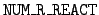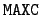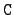Next: The array ident_data Up: Structures identifying the data Previous: The array i_react_data   Contents

## The array r_react_data

This array contains the real-number information about a target. The dimensions of the array are r_react_data(NUM_R_REACT, MAXC), with the values= 6 and= 200. This array is reset for each new target. Its second index ranges over the reactions C for the target, and for each reaction the first index identifies the following items.

r_react_data(1, c_count):
The energy of the reaction, q0. For= 5 this value is zero, and an array of flux-weighted bin averages Qg is computed.

r_react_data(2, c_count):
The first extra information, x1. The content of this variable is determined by the value of S as explained in Section 2.3

r_react_data(3, c_count):
The second extra information, x2. The meaning of this variable is also determined by the value of the S reaction identifier.

r_react_data(4, c_count):
The calculated reaction threshold. This number is calculated by the subroutine parts and is based on the mass balance. It is not used now, but it is available.

r_react_data(5, c_count):
The minimum energy for the reaction Emin. This number is printed out only for gamma production reactions.

r_react_data(6, c_count):
The maximum energy for the reaction Emax. This number is also printed out only for gamma production reactions.Next: The array ident_data Up: Structures identifying the data Previous: The array i_react_data   Contents